# sklearn.model_selection.train_test_split¶

sklearn.model_selection.train_test_split(*arrays, **options)[source]

Split arrays or matrices into random train and test subsets

Quick utility that wraps input validation and next(ShuffleSplit().split(X, y)) and application to input data into a single call for splitting (and optionally subsampling) data in a oneliner.

Read more in the User Guide.

Parameters: *arrays : sequence of indexables with same length / shape Allowed inputs are lists, numpy arrays, scipy-sparse matrices or pandas dataframes. test_size : float, int or None, optional (default=None) If float, should be between 0.0 and 1.0 and represent the proportion of the dataset to include in the test split. If int, represents the absolute number of test samples. If None, the value is set to the complement of the train size. If train_size is also None, it will be set to 0.25. train_size : float, int, or None, (default=None) If float, should be between 0.0 and 1.0 and represent the proportion of the dataset to include in the train split. If int, represents the absolute number of train samples. If None, the value is automatically set to the complement of the test size. random_state : int, RandomState instance or None, optional (default=None) If int, random_state is the seed used by the random number generator; If RandomState instance, random_state is the random number generator; If None, the random number generator is the RandomState instance used by np.random. shuffle : boolean, optional (default=True) Whether or not to shuffle the data before splitting. If shuffle=False then stratify must be None. stratify : array-like or None (default=None) If not None, data is split in a stratified fashion, using this as the class labels. splitting : list, length=2 * len(arrays) List containing train-test split of inputs. New in version 0.16: If the input is sparse, the output will be a scipy.sparse.csr_matrix. Else, output type is the same as the input type.

Examples

>>> import numpy as np
>>> from sklearn.model_selection import train_test_split
>>> X, y = np.arange(10).reshape((5, 2)), range(5)
>>> X
array([[0, 1],
[2, 3],
[4, 5],
[6, 7],
[8, 9]])
>>> list(y)
[0, 1, 2, 3, 4]

>>> X_train, X_test, y_train, y_test = train_test_split(
...     X, y, test_size=0.33, random_state=42)
...
>>> X_train
array([[4, 5],
[0, 1],
[6, 7]])
>>> y_train
[2, 0, 3]
>>> X_test
array([[2, 3],
[8, 9]])
>>> y_test
[1, 4]

>>> train_test_split(y, shuffle=False)
[[0, 1, 2], [3, 4]]


## Examples using sklearn.model_selection.train_test_split¶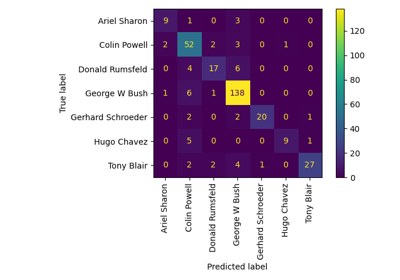Faces recognition example using eigenfaces and SVMs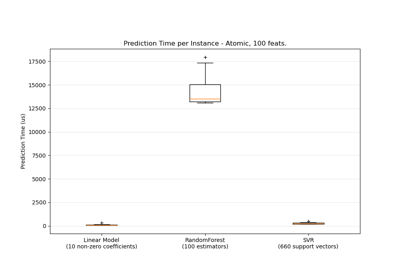Prediction Latency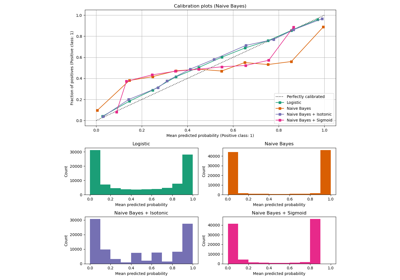Probability Calibration curves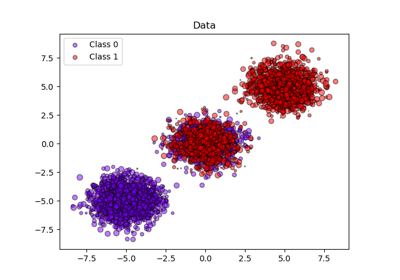Probability calibration of classifiers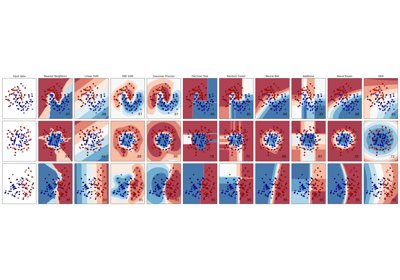Classifier comparisonColumn Transformer with Mixed Types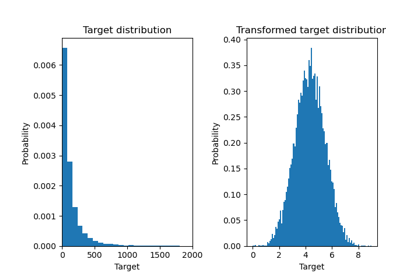Effect of transforming the targets in regression model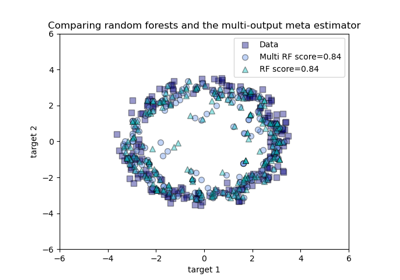Comparing random forests and the multi-output meta estimator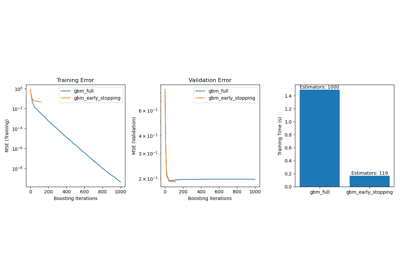Early stopping of Gradient Boosting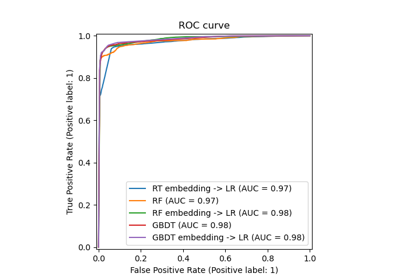Feature transformations with ensembles of trees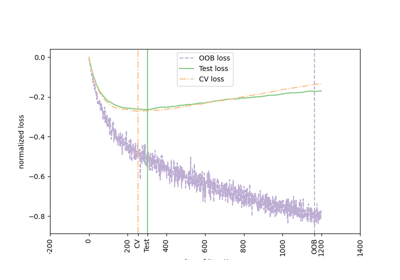Gradient Boosting Out-of-Bag estimatesPipeline Anova SVMComparing various online solversMNIST classfification using multinomial logistic + L1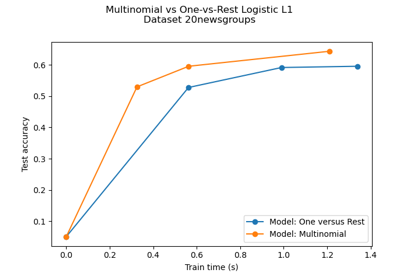Multiclass sparse logisitic regression on newgroups20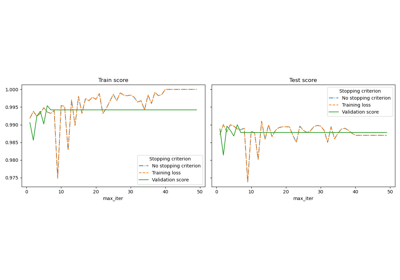Early stopping of Stochastic Gradient DescentParameter estimation using grid search with cross-validation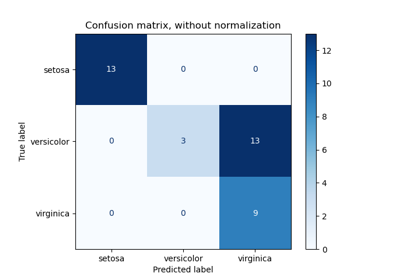Confusion matrix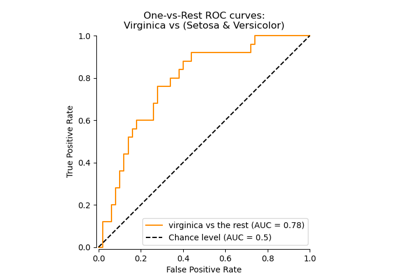Receiver Operating Characteristic (ROC)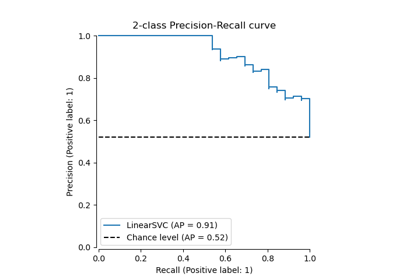Precision-Recall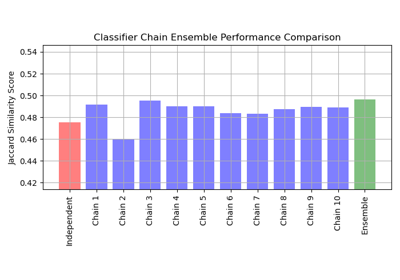Classifier Chain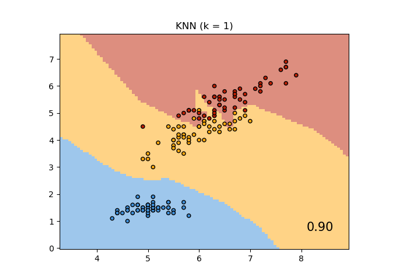Comparing Nearest Neighbors with and without Neighborhood Components Analysis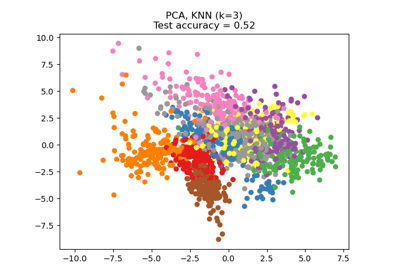Dimensionality Reduction with Neighborhood Components Analysis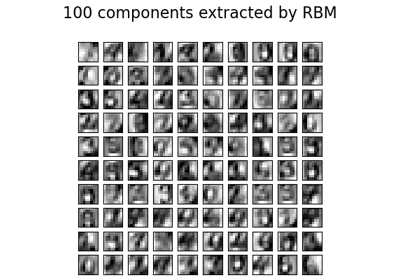Restricted Boltzmann Machine features for digit classification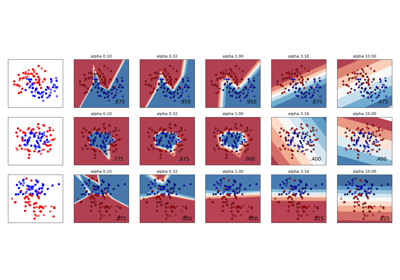Varying regularization in Multi-layer PerceptronUsing FunctionTransformer to select columns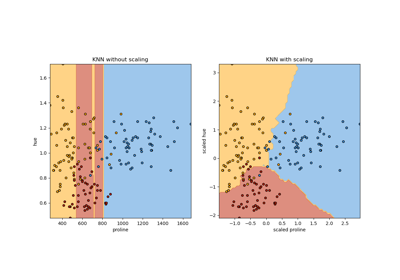Importance of Feature Scaling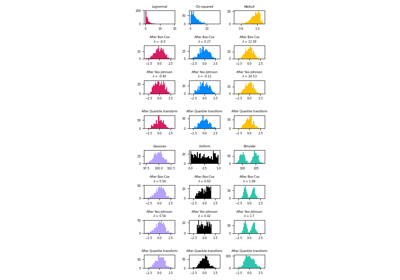Map data to a normal distribution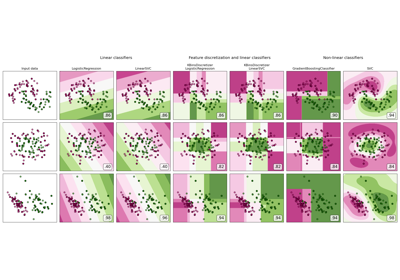Feature discretization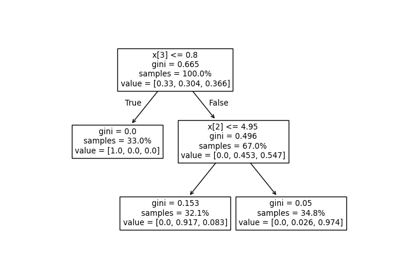Understanding the decision tree structure